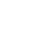# Mathematics research

Massey University has some of the highest-ranked mathematics researchers in New Zealand. We conduct research in analysis and number theory, industrial and applied mathematics, mathematical biology, numerical analysis, combinatorics and topology, and mathematical physics.

## Research expertise

### Applied mathematics

Our research combines diverse aspects of modelling and analysis, including developing and studying mathematical models of varied applications including blood flow, powder flow and hydrothermal eruptions.

We work on industrial problems involving physical systems and transport processes.

### Differential equations

Differential equations are used in a wide range of applications. Our researchers focus on studying ordinary, partial, stochastic, delayed, discontinuous and non-local differential equations, and solving them analytically and numerically. We also have expertise in geometric integration and domain decomposition methods.

### Dynamical systems

We are advancing theoretical aspects of dynamical system including developing and using multiscale methods and dimension reduction techniques for complex dynamical systems.

We also study applications including stellar dynamics and celestial mechanics, networks of coupled oscillators and cardio-respiratory system function.

### Geometry and analysis

We study non-linear elasticity, materials science and low dimensional topology and geometry, particularly hyperbolic geometry, discrete groups and their associated universal constants, and relations between arithmetic and geometry.

### Mathematical modelling

We model continuum mechanics, flow in porous media, heat and mass transfer, geophysical systems and pollution transport, stellar dynamics and celestial mechanics, breathing and gas exchange and the spread of infectious diseases.

### Mathematical physics

We are using powerful mathematical techniques to investigate problems in physics, biophysics and interdisciplinary areas. The main focus is on theoretical particle physics, particularly the quark structure of matter and the properties of neutrinos. We also have expertise in random matrices.

### Number theory, combinatorics, special functions and topology

We study special functions' properties, especially elliptic and theta functions and modular forms, and their applications in number theory and combinatorics.

Research focuses on the application of combinatorial techniques to a wide range of problems, including links in three and higher dimensions.

## Study

Find programmes with a research element, including the PhD.## Search for an expert

Search our staff database for an expert or area of expertise.

## You may also be interested in research in

Massey Contact Centre Mon - Fri 8:30am to 4:30pm 0800 MASSEY (+64 6 350 5701) TXT 5222 contact@massey.ac.nz Web chat Staff Alumni News Māori @ Massey